# Mathematics

(Redirected from New Math)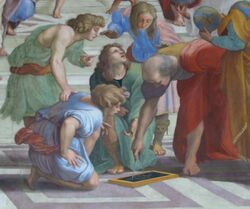Euclid, Greek mathematician, 3rd century BC, giving what may be the first ever PowerPoint presentation

Mathematics (from Greek άθημα máthēma, “knowledge, study, learning”) is the systematic torture of students disguised as the study of quantity, structure, space, and change. Mathematicians seek out patterns and formulate new conjectures, trying to make sense of the universe by examining these abstract concepts, determining common traits, and then experimenting to see if this advanced knowledge will help them get women into bed. These conjectures are often faulty, and this subsequent failure is referred to as mathematical proof.

Galileo Galilei (1564-1642) said, "The universe cannot be read until we have learned the language and become familiar with the characters in which it is written. It is written in mathematical language... Without these, one is wandering about in a dark labyrinth". He was also condemned by the church for heresy, and the only surviving body part of his is his middle finger on his right hand. This is currently kept at the Museo Galileo in Florence, Italy, allowing him to give the finger to the Catholic Church from beyond the grave.

## Etymology

The word "mathematics" comes from the Greek μάθημα (máthēma), which means in ancient Greek what one learns, what one gets to know, hence also study and science, and in modern Greek just lesson. Which means that the Greek translation of “Studying science in a mathematics lesson” is “μάθημα μάθημα μάθημα μάθημα”. Which shows why Greek philosophers appear to be so intelligent - they can simply say the same word half a dozen times and nobody knows what they really mean.

In Latin, and in English until around 1700, the term "mathematics" more commonly meant "astrology" (or sometimes "astronomy") rather than "mathematics"; the meaning gradually changed to its present one from about 1500 to 1800. This has resulted in several mistranslations: a particularly notorious one is Saint Augustine's warning that Christians should beware of "mathematici" meaning mathematicians, which is sometimes mistranslated as a condemnation of astrologers. Given that the three Biblical magi that visited Jesus in the manger were most likely astrologers, this is obviously not the case.

In English, the noun mathematics takes singular verb forms. It is often shortened to maths or, in English-speaking North America, math, because they are too lazy to learn how to rite goodly.

## Inspiration, pure and applied mathematics, and aesthetics

For those who are mathematically inclined, there is often a definite aesthetic aspect to much of mathematics. Many mathematicians talk about the elegance of mathematics, its intrinsic aesthetics and inner beauty. Simplicity and generality are valued. There is beauty in a simple and elegant proof. G. H. Hardy expressed the belief that these aesthetic considerations are, in themselves, sufficient to justify the study of pure mathematics. He identified criteria such as significance, unexpectedness, inevitability, and economy as factors that contribute to a mathematical aesthetic. The popularity of recreational mathematics is another sign of the pleasure many find in solving mathematical questions.

This of course can be taken as further proof that advanced skills in mathematics do not lead to a healthy and active social life, and mathematicians need to get their kicks wherever they can.

## Notation, language, and rigor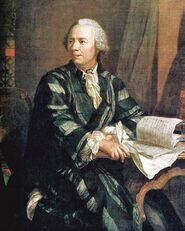Euler, who simplified mathematical notation by making us learn a completely new language.

Most of the mathematical notation in use today was not invented until the 16th century. Before that, mathematics was written out in words, a painstaking process that limited mathematical discovery. For example, π was written as three decimal point one four one five nine two six five three five and lots of other numbers.

Euler was responsible for many of the notations in use today. Like musical notation, modern mathematical notation has a strict syntax, which may make it hard for beginners to understand — which is surprising, as drummers appear to understand musical notation, and yet often have difficulty finding their own arse without the aid of a map, a compass, and the assistance of at least three roadies.

Mathematical language can be difficult to understand for beginners. Technical terms such as homeomorphism have precise meanings in mathematics. Mathematicians refer to this precision of language and logic as "rigor"; however, there is a suspicion that they are just making shit up to confuse everyone else. This is yet another indicator of their desperate need to copulate with a flesh-and-blood individual.

### Basic Notation

 + Plus Put more on - Minus Take it off = Double Minus Take it all off ± Plus Minus Take it both ways / Slash Guitarist ** Star Reward for a job well done $x$Ex Alimony payments are due > Down Go down stairs < Up Go up stairs $\pi$Pi Apple for preference ln() Natural log A fallen tree log() Log rhythm A tree cut down $e$Natural base The bit of the fallen tree facing down Duraflame Unnatural log Sawdust and paraffin sin() Sin Why the church hates mathematics cos() Cos A type of lettuce tan() Tan Get some sun. $i$${\sqrt {-1}}$Imaginary number, named after the inventor's girlfriend

## Fields of mathematics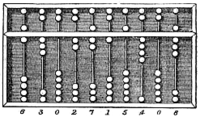An abacus. This was an early form of iPad, but with better battery life

Mathematics can, broadly speaking, be subdivided into the study of quantity, structure, space, and change (i.e. arithmetic, algebra, geometry, and analysis).

These divisions are broad generalities, and the scope, nature, and even the number of divisions is a topic debated by mathematicians. These debates can become extremely agitated with mathematicians becoming wide-eyed and foaming at the mouth with passion, which is symptomatic of a group of people with no sexual outlet.

### Fundamentals of Mathematics

Two major elements of the foundations of mathematics are mathematical logic, set theory, and category theory. The crisis of foundations was stimulated by a number of controversies, including the controversy over Cantor's set theory, which was eventually resolved by having the cutlery for the first courses on the outside and gradually moving in each course. Also controversial was the Brouwer-Hilbert controversy, which has been resolved in that most mathematicians use brown ketchup instead of red with lobster. Given they don't get much sex, mathematicians are very particular about their degustationary arts.

Mathematical logic is concerned with setting mathematics within a rigorous axiomatic framework and whipping them until they cry out for their mothers.

Theoretical computer science is the study of mathematics as it relates to a computing or calculatory system. A classic example of this is the Turing Duck Test, where it can be determined that a member of the anatidae family may resemble a hominidae to the observer if the hominidae has had artificial depressants. Further testing has revealed that excessive depressant use by the observer will increase the resemblance between the two. It will also create feelings of arousal in the observer directed toward the anatidae, although said observer is generally a mathematician, and due to the aforementioned lack of recreational sex by the observer at this stage a cactaceae would be a veritable succubus.

 $p\Rightarrow q\,$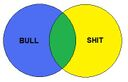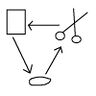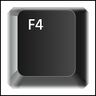Mathematical logic Set theory Category theory Theory of computation

### Pure mathematics

#### Quantity

The study of quantity starts with numbers, first the familiar natural numbers and integers ("whole numbers") and arithmetical operations on them, which are characterized in arithmetic. You would probably have assumed that all mathematics comes back to numbers, but mathematics is hard.

As the number system is further developed, the integers are recognized as a subset of the rational numbers. The positive side of rational numbers is that they don't get jealous when you use other numbers, or do irrational things like adding a red sock when you're washing whites.

These, in turn, are contained within the real numbers, which are used to represent continuous quantities. Real numbers are completely unlike those false numbers that say that they care about you, but then go running around with engineers or pharmacologists as soon as you turn back to your whiteboard.

Real numbers are generalized to complex numbers. Complex numbers are to natural numbers what Chernobyl wheat is to organic carrots. These are the numbers that will tell you any number of pretty lies, wait until you've let your guard down, and then "borrow" your money or your car and run off to Las Vegas to be a cocktail hostess.

 $1,2,3\,...\!$$...-2,-1,0,1,2\,...\!$$-2,{\frac {2}{3}},1.21\,\!$$-e,{\sqrt {2}},3,\pi \,\!$$2,i,-2+3i,2e^{i{\frac {4\pi }{3}}}\,\!$Natural numbers Integers Rational numbers Real numbers Complex numbers

#### Structure

Many mathematical objects, such as sets, types, or functions, consist of additional mathematical objects that in some manner attach (or relate) to the set. This has very little to do with architectural structure, so it is suggested that you avoid builders who use this form of mathematics, unless you prefer to have your roof laid vertically.

By its generality, abstract algebra can often be applied to seemingly unrelated problems, including a number of ancient problems concerning compass and straightedge constructions, like the Delian problem. This problem came initially from the Oracle at Delphi, who told the Greeks that Apollo would only save them from a nasty plague if they could build a new altar exactly double the volume of the original one, using only a compass and a ruler.

Using the theory of fields, it was proved in 1837 that creating a double-sized altar using just a ruler and compass is impossible. Which suggests that the Oracle, like many women, was actually a hard-hearted bitch.

 ${\begin{matrix}(1,2,3)&(1,3,2)\\(2,1,3)&(2,3,1)\\(3,1,2)&(3,2,1)\end{matrix}}$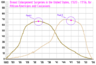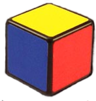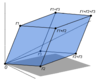Combinatorics Number theory Group theory Graph theory Order theory

#### Space

Space, the final mathematical frontier. This is the study of Euclidean geometry. To seek out new angles and new dimensions. To boldly plot what has never been plotted before.

Although in actuality mathematics does go beyond space, so as such calling it the "final frontier" is somewhat erroneous.

Space mathematics is all about angles and shapes. The Pythagorean theorem is at its fore, where the square of the hypotenuse is equal to the sum of the squares of its other two sides, or a2 + b2 = c2. This, of course, relates to a right-angled triangle, and is not to ever be confused for a circle, which has a completely different formula to determine. And there are no exceptions, except in spherical geometry. Or hyperbolic geometry. Or in three-dimensional space.

Other than those few minor exceptions, the Pythagorean theorem is 100% effective with absolutely no exceptions.

Except differential geometry.

#### Change

One of the most exciting fields of mathematics, calculus is the beginning of the study of change. However, it has branched off into a significant number of areas, including string theory, quantum mechanics, and other areas.

The science of quantum mechanics leads itself into trying to directly understand exactly what the universe is made up of and how it works. This has created an entirely new field of mathematics known as uncertainty that has led mathematicians to realize that we cannot understand anything as a complete certainty. We cannot know exactly where an object is, what it is, how much it weighs, what it tastes like, or even if it exists at all.

Plato once stated "True knowledge comes from knowing that you know nothing." This is uncertainty principle in it purest form, written around two-and-a-half thousand years ago, so it's not exactly a startling new revelation. But mathematics is often about proving again what was proven before, and "just knowing" something is right or wrong does not count as proof.

Therefore, in short, most mathematics is a waste of time.

## Intellectual safety levels

To protect the general public from potentially hazardous ideas, The United States Center for Cleverness Control and Prevention has classified various disciplines in levels of intellectual hazard, Level 1 being the minimal risk and Level 5 being the maximal risk.

• Intellectual Hazard Level 1: The study of successor functions and inductive sets
• Intellectual Hazard Level 2: Studying the image Frac(Z); Exponentiation; Study of the rings R[X].
• Intellectual Hazard Level 3: Game theory; Stochastics; Calculus; Number theory; Ordinary Differential Equations; Combinatorics.
• Intellectual Hazard Level 4: Studying functions of complex numbers; Defining what it means to be "near" something else; Logic; Algebra (such as symmetries of squares); Analysis of things which change sets of points; Calculus of things where the end result changes a lot.
• Intellectual Hazard Level 5: Talking about sets; Drawing pictures on surfaces; Studying curves; Relating slopes with functions; Functions acting on donuts; Studying why functions can't be extended to bigger sets; String theory.

While this is often used as a baseline for determining the risk of dealing with various types of mathematics, it is not an exhaustive list. Fields such as the Riemann hypothesis should never be attempted without significant safety precautions.

## Mathematics as profession

Arguably the most prestigious award in mathematics is the Fields Medal, established in 1936, and now awarded every 4 years. The Fields Medal is often considered a mathematical equivalent to the Nobel Prize, mainly because nobody at the Royal Swedish Academy of Sciences thinks mathematics is prize-worthy.

A list of seven important problems, titled the "Millennium Prize Problems", was published in 2000. The solution of each of these problems carries a $1 million reward. One such question is why it pays out$1 million when Millennium relates to one thousand.

Beyond this, there is not much that is profitable in mathematics. Most mathematicians take up places in institutes of learning, teaching budding mathematicians the skills that made them so ineffectual.

But most importantly, professional mathematicians never get any sex. Ever. So when your guidance counsellor suggests you consider a field in mathematics, you'll be looking at a long, lonely road of division, subtracted from the mainstream of society. What this all adds up to... is that mathematicians never multiply.

## Footnotes

1. 1564 - 1642 = -78
2. c570 - c495 = c75
3. Don't worry, he's not important. You won't be quizzed on this later.
4. Advanced mathematicians don't strain themselves by doing anything as mundane as counting to three.
5. And they still won't sleep with you!
6. Which has nothing to do with agriculture.
7. a2 + b2 = c2
8. As stated before, due to their predominantly asexual nature, what excites a mathematician is not necessarily what would excite most people.
9. Also referred to as non-Euclidiean geometry. We're not actually referring to graffiti here at all. That comes under fluid dynamics.
10. Being arithmetically correct is so mundane.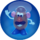Featured Article  (read another featured article) Featured version: 29 March 2012 This article has been featured on the main page. — You can vote for or nominate your favourite articles at Uncyclopedia:VFH.